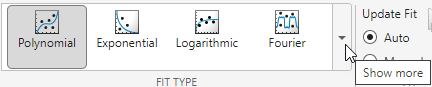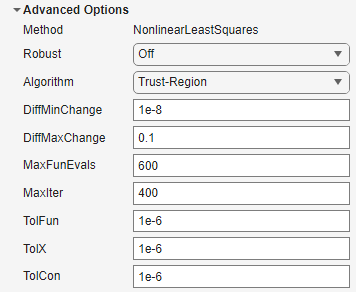## Parametric Fitting

### Parametric Fitting with Library Models

Parametric fitting involves finding coefficients (parameters) for one or more models that you fit to data. The data is assumed to be statistical in nature and is divided into two components:

data = deterministic component + random component

The deterministic component is given by a parametric model and the random component is often described as error associated with the data:

data = parametric model + error

The model is a function of the independent (predictor) variable and one or more coefficients. The error represents random variations in the data that follow a specific probability distribution (usually Gaussian). The variations can come from many different sources, but are always present at some level when you are dealing with measured data. Systematic variations can also exist, but they can lead to a fitted model that does not represent the data well.

The model coefficients often have physical significance. For example, suppose you collected data that corresponds to a single decay mode of a radioactive nuclide, and you want to estimate the half-life (T1/2) of the decay. The law of radioactive decay states that the activity of a radioactive substance decays exponentially in time. Therefore, the model to use in the fit is given by

`$y={y}_{0}{e}^{-\lambda t}$`

where y0 is the number of nuclei at time t = 0, and λ is the decay constant. The data can be described by

`$\text{data}={y}_{0}{e}^{-\lambda t}+\text{error}$`

Both y0 and λ are coefficients that are estimated by the fit. Because T1/2 = ln(2)/λ, the fitted value of the decay constant yields the fitted half-life. However, because the data contains some error, the deterministic component of the equation cannot be determined exactly from the data. Therefore, the coefficients and half-life calculation will have some uncertainty associated with them. If the uncertainty is acceptable, then you are done fitting the data. If the uncertainty is not acceptable, then you might have to take steps to reduce it either by collecting more data or by reducing measurement error and collecting new data and repeating the model fit.

With other problems where there is no theory to dictate a model, you might also modify the model by adding or removing terms, or substitute an entirely different model.

The Curve Fitting Toolbox™ parametric library models are described in the following sections.

### Select Model Type

#### Select Model Type Interactively

Open the Curve Fitter app by entering `curveFitter` at the MATLAB® command line. Alternatively, on the Apps tab, in the Math, Statistics and Optimization group, click Curve Fitter.

In the Curve Fitter app, go to the Fit Type section of the Curve Fitter tab. You can select a model type from the fit gallery. Click the arrow to open the gallery.This table describes the models that you can fit for curves and surfaces.

Fit GroupFit CategoryCurvesSurfaces
Regression ModelsPolynomialYes (up to degree 9)Yes (up to degree 5)
ExponentialYesNo
FourierYesNo
GaussianYesNo
PowerYesNo
RationalYesNo
Sum of SineYesNo
WeibullYesNo
InterpolationInterpolant

Yes, with methods:

• Nearest neighbor

• Linear

• Cubic

• Shape-preserving (PCHIP)

Yes, with methods:

• Nearest neighbor

• Linear

• Cubic

• Biharmonic

• Thin-plate spline

SmoothingSmoothing SplineYesNo
LowessNoYes
CustomCustom EquationYesYes
Custom Linear FittingYesNo

The Results pane displays the model specifications, coefficient values, and goodness-of-fit statistics.

Tip

The Curve Fitter app provides a selection of fit types and settings in the Fit Options pane that you can change to try to improve your fit. Try the defaults first, and then experiment with other settings. For more details on how to use the available fit options, see Specify Fit Options and Optimized Starting Points.

You can try a variety of settings for a single fit and you can create multiple fits to compare. When you create multiple fits in the Curve Fitter app, you can compare different fit types and settings side by side. For more information, see Create Multiple Fits in Curve Fitter App.

#### Select Model Type Programmatically

You can specify a library model name as a character vector or string scalar when you call the `fit` function. For example, you can specify a quadratic `poly2` model:

`f = fit(x,y,"poly2")`

To view all available library model names, see List of Library Models for Curve and Surface Fitting to view all available library model names.

You can also use the `fittype` function to construct a `fittype` object for a library model, and use the `fittype` as an input to the `fit` function.

Use the `fitoptions` function to find out what parameters you can set, for example:

`fitoptions(poly2)`

For examples, see the sections for each model type, listed in the table in Select Model Type Interactively. For details on all the functions for creating and analysing models, see Curve and Surface Fitting.

### Center and Scale Data

Most fits in the Curve Fitter app provide the Center and scale option in the Fit Options pane. When you select this option, the app refits the model with the data centered and scaled. At the command line, use the `fitoptions` function with the `Normalize` option set to `'on'`.

To alleviate numerical problems with variables of different scales, normalize the input data (also known as predictor data). For example, suppose your surface fit inputs are engine speed with a range of 500–4500 r/min and engine load percentage with a range of 0–1. Then, Center and scale generally improves the fit because of the great difference in scale between the two inputs. However, if your inputs are in the same units or similar scale (for example, eastings and northings for geographic data), then Center and scale is less useful. When you normalize inputs with this option, the values of the fitted coefficients change when compared to the original data.

If you are fitting a curve or surface to estimate coefficients, or the coefficients have physical significance, clear the Center and scale check box. The plots in the Curve Fitter app always use the original scale, regardless of the Center and scale status.

At the command line, to center and scale the data before fitting, create the `options` structure by using the `fitoptions` function with `options.Normal` specified as `'on'`. Then, use the `fit` function with the specified options.

```options = fitoptions; options.Normal = 'on'; options options = Normalize: 'on' Exclude: [1x0 double] Weights: [1x0 double] Method: 'None' load census f1 = fit(cdate,pop,"poly3",options)```

### Specify Fit Options and Optimized Starting Points

#### Fit Options in Curve Fitter App

In the Curve Fitter app, you can specify fit options interactively in the Fit Options pane. All fits except Interpolant and Smoothing Spline have configurable fit options. The available options depend on the fit you select (that is, linear, nonlinear, or nonparametric fit).

• The options described here are available for nonlinear models.

• Lower and Upper coefficient constraints are the only fit options available in the Fit Options pane for Polynomial fits.

• Nonparametric fits (that is, Interpolant, Smoothing Spline, and Lowess fits) do not have Advanced Options.

The Fit Options pane for the single-term Exponential fit is shown here. The Coefficient Constraints values are for the `census` data.For more information about these fit options, see the `lsqcurvefit` (Optimization Toolbox) function.

#### Optimized Starting Points and Default Constraints

The default coefficient starting points and constraints for fits in the Fit Type pane are shown in the following table. If the starting points are optimized, then they are calculated heuristically based on the current data set. Random starting points are defined on the interval [0 1] and linear models do not require starting points. If a model does not have constraints, the coefficients have neither a lower bound nor an upper bound. You can override the default starting points and constraints by providing your own values in the Fit Options pane.

Fit

Starting Points

Constraints

```Linear Fitting```

N/A

None

```Custom Equation```

Random

None

`Exponential`

Optimized

None

`Fourier`

Optimized

None

`Gaussian`

Optimized

ci > 0

`Polynomial`

N/A

None

`Power`

Optimized

None

`Rational`

Random

None

```Sum of Sine```

Optimized

bi > 0

`Weibull`

Random

a, b > 0

The Sum of Sine and Fourier fits are particularly sensitive to starting points, and the optimized values might be accurate for only a few terms in the associated equations.

#### Specify Fit Options at the Command Line

Create the default fit options structure and set the option to center and scale the data before fitting:

```options = fitoptions; options.Normal = 'on'; options options = Normalize: 'on' Exclude: [1x0 double] Weights: [1x0 double] Method: 'None'```

Modifying the default fit options structure is useful when you want to set the `Normalize`, `Exclude`, or `Weights` fields, and then fit your data using the same options with different fitting methods. For example:

```load census f1 = fit(cdate,pop,"poly3",options); f2 = fit(cdate,pop,"exp1",options); f3 = fit(cdate,pop,"cubicsp",options);```

Data-dependent fit options are returned in the third output argument of the `fit` function. For example, the smoothing parameter for smoothing spline is data-dependent:

```[f,gof,out] = fit(cdate,pop,"smooth"); smoothparam = out.p smoothparam = 0.0089```

Use fit options to modify the default smoothing parameter for a new fit:

```options = fitoptions("Method","Smooth","SmoothingParam",0.0098); [f,gof,out] = fit(cdate,pop,"smooth",options);```

For more details on using fit options, see the `fitoptions` function.